# What Is A Heap Data Structure In Java

This tutorial explains what is Java Heap Data Structure & related concepts such as Min Heap, Max Heap, Heap Sort, Stack vs Heap with examples:

A heap is a special data structure in Java. A heap is a tree-based data structure and can be classified as a complete binary tree. All the nodes of the heap are arranged in a specific order.

• Heap Data Structure In Java
• Binary Heap
• Min Heap In Java
• Min Heap Algorithm
• Min Heap Implementation In Java
• Max Heap In Java
• Priority Queue Min Heap In Java
• Priority Queue Max Heap In Java
• Heap Sort In Java
• Heap Sort Algorithm In Java
• Heap Sort Implementation In Java
• Stack Vs Heap In Java
• Frequently Asked Questions
• Conclusion
• Recommended Reading

## Heap Data Structure In Java

In the heap data structure, the root node is compared with its children and arranged according to the order. So if a is a root node and b is its child, then the property, key (a)>= key (b) will generate a max heap.

The above relation between the root and the child node is called as “Heap Property”.

Depending on the order of parent-child nodes, the heap is generally of two types:

#1) Max-Heap: In a Max-Heap the root node key is the greatest of all the keys in the heap. It should be ensured that the same property is true for all the subtrees in the heap recursively.

The below diagram shows a Sample Max Heap. Note that the root node is greater than its children.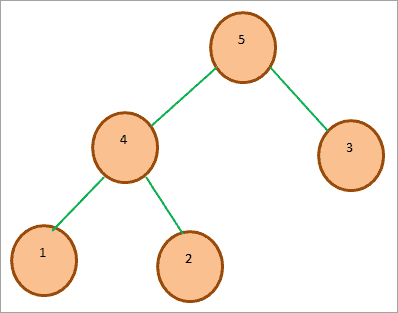#2) Min-Heap: In the case of a Min-Heap, the root node key is the smallest or minimum among all the other keys present in the heap. As in Max heap, this property should be recursively true in all the other subtrees in the heap.

An example, of a Min-heap tree, is shown below. As we can see, the root key is the smallest of all the other keys in the heap.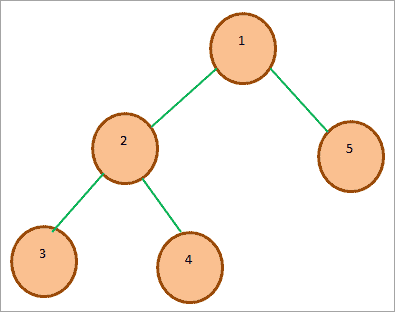A heap data structure can be used in the following areas:

• Heaps are mostly used to implement Priority Queues.
• Especially min-heap can be used to determine the shortest paths between the vertices in a Graph.

As already mentioned, the heap data structure is a complete binary tree that satisfies the heap property for root and the children. This heap is also called as a binary heap.

### Binary Heap

A binary heap fulfills the below properties:

• A binary heap is a complete binary tree. In a complete binary tree, all the levels except the last level are completely filled. At the last level, the keys are as far as left as possible.
• It satisfies the heap property. The binary heap can be max or min-heap depending on the heap property it satisfies.

A binary heap is normally represented as an Array. As it is a complete binary tree, it can easily be represented as an array. Thus in an array representation of binary heap, the root element will be A where A is the array used to represent the binary heap.

So in general for any ith node in the binary heap array representation, A[i], we can represent the indices of other nodes as shown below.

A [(i-1)/2]Represents the parent node
A[(2*i)+1]Represents the left child node
A[(2*i)+2]Represents the right child node

Consider the following binary heap: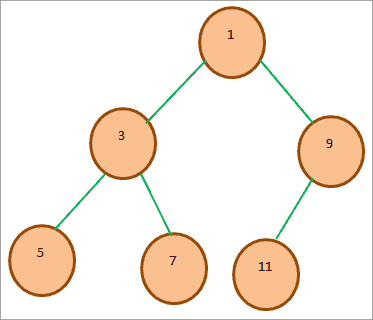The array representation of the above min binary heap is as follows: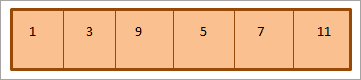As shown above, the heap is traversed as per the level order i.e. the elements are traversed from left to right at each level. When the elements at one level are exhausted, we move on to the next level.

Next, we will implement the binary heap in Java.

The below program demonstrates the binary heap in Java.

 `import` `java.util.*;``class` `BinaryHeap {``private` `static` `final` `int` `d= ``2``;``private` `int``[] heap;``private` `int` `heapSize;``//BinaryHeap constructor with default size``public` `BinaryHeap(``int` `capacity){``heapSize = ``0``;``heap = ``new` `int``[ capacity+``1``];``Arrays.fill(heap, -``1``);``}``//is heap empty?``public` `boolean` `isEmpty(){``return` `heapSize==``0``;``}``//is heap full?``public` `boolean` `isFull(){``return` `heapSize == heap.length;``}``//return parent``private` `int` `parent(``int` `i){``return` `(i-``1``)/d;``}``//return kth child``private` `int` `kthChild(``int` `i,``int` `k){``return` `d*i +k;``}``//insert new element into the heap``public` `void` `insert(``int` `x){``if``(isFull())``throw` `new` `NoSuchElementException(``"Heap is full, No space to insert new element"``);``heap[heapSize++] = x;``heapifyUp(heapSize-``1``);``}``//delete an element from the heap at given position``public` `int` `delete(``int` `x){``if``(isEmpty())``throw` `new` `NoSuchElementException(``"Heap is empty, No element to delete"``);``int` `key = heap[x];``heap[x] = heap[heapSize -``1``];``heapSize--;``heapifyDown(x);``return` `key;``}``//maintain heap property during insertion``private` `void` `heapifyUp(``int` `i) {``int` `temp = heap[i];``while``(i>``0` `&& temp > heap[parent(i)]){``heap[i] = heap[parent(i)];``i = parent(i);``}``heap[i] = temp;``}``//maintain heap property during deletion``private` `void` `heapifyDown(``int` `i){``int` `child;``int` `temp = heap[i];``while``(kthChild(i, ``1``) < heapSize){``child = maxChild(i);``if``(temp < heap[child]){ heap[i] = heap[child]; }``else` `break``; i = child; } heap[i] = temp; } ``private` `int` `maxChild(``int` `i) { ``int` `leftChild = kthChild(i, ``1``); ``int` `rightChild = kthChild(i, ``2``); ``return` `heap[leftChild]>heap[rightChild]?leftChild:rightChild;``}``//print the heap``public` `void` `printHeap()``{``System.out.print(``"nHeap = "``);``for` `(``int` `i = ``0``; i < heapSize; i++)``System.out.print(heap[i] +``" "``);``System.out.println();``}``//return max from the heap``public` `int` `findMax(){``if``(isEmpty())``throw` `new` `NoSuchElementException(``"Heap is empty."``);``return` `heap[``0``];``}``}``class` `Main{``public` `static` `void` `main(String[] args){``BinaryHeap maxHeap = ``new` `BinaryHeap(``10``);``maxHeap.insert(``1``);``maxHeap.insert(``2``);``maxHeap.insert(``3``);``maxHeap.insert(``4``);``maxHeap.insert(``5``);``maxHeap.insert(``6``);``maxHeap.insert(``7``);``maxHeap.printHeap();``//maxHeap.delete(5);``//maxHeap.printHeap();``}``}`

Output:

nHeap = 7 4 6 1 3 2 5

### Min Heap In Java

A min-heap in Java is a complete binary tree. In min-heap, the root node is smaller than all the other nodes in the heap. In general, the key value of each internal node is smaller than or equal to its child nodes.

As far as array representation of min-heap is concerned, if a node is stored at position ‘i’, then its left child node is stored at position 2i+1 and then the right child node is at position 2i+2. The position (i-1)/2 returns its parent node.

Enlisted below are the various operations supported by min-heap.

#1) Insert (): Initially, a new key is added at the end of the tree. If the key is larger than its parent node, then the heap property is maintained. Otherwise, we need to traverse the key upwards to fulfill the heap property. Insertion operation in min heap takes O (log n) time.

#2) extractMin (): This operation removes the minimum element from the heap. Note that the heap property should be maintained after removing the root element (min element) from the heap. This entire operation takes O (Logn).

#3) getMin (): getMin () returns the root of the heap which is also the minimum element. This operation is done in O (1) time.

Given below is an example tree for a Min-heap.The above diagram shows a min-heap tree. We see that the root of the tree is the minimum element in the tree. As the root is at location 0, its left child is placed at 2*0 + 1 = 1 and right child is at 2*0 + 2 = 2.

#### Min Heap Algorithm

Given below is the algorithm for building a min-heap.

 `procedure build_minheap ``Array Arr: of size N => array of elements``    ``{``        ``repeat ``for` `(i = N/``2` `; i >= ``1` `; i--)``            ``call procedure min_heapify (A, i);``    ``}``procedure min_heapify (var A[ ] , var i, var N)``    ``{``    ``var left  = ``2``*i;``    ``var right = ``2``*i+``1``;``    ``var smallest;``    ``if``(left <= N and A[left] < A[ i ] )``         ``smallest = left;``    ``else``        ``smallest = i;``    ``if``(right <= N and A[right] < A[smallest] )``        ``smallest = right;``    ``if``(smallest != i)``    ``{``        ``swap A[ i ] and  A[ smallest ]);``        ``call min_heapify (A, smallest,N);``    ``} ``    ``}`

#### Min Heap Implementation In Java

We can implement the min-heap either using array or priority queues. Implementing min-heap using priority queues is the default implementation as a priority queue is implemented as min-heap.

The following Java program implements the min-heap using Arrays. Here we use array representation for heap and then apply heapify function to maintain the heap property of each element added to the heap. Finally, we display the heap.

 `class` `Min_Heap { ``    ``private` `int``[] HeapArray; ``    ``private` `int` `size; ``    ``private` `int` `maxsize; ``  ``    ``private` `static` `final` `int` `FRONT = ``1``; ``  ``    ``//constructor to initialize the HeapArray``    ``public` `Min_Heap(``int` `maxsize)  { ``        ``this``.maxsize = maxsize; ``        ``this``.size = ``0``; ``        ``HeapArray = ``new` `int``[``this``.maxsize + ``1``]; ``        ``HeapArray[``0``] = Integer.MIN_VALUE; ``    ``} ``  ``    ``// returns parent position for the node ``    ``private` `int` `parent(``int` `pos)  { ``        ``return` `pos / ``2``; ``    ``} ``  ``    ``// returns the position of left child ``    ``private` `int` `leftChild(``int` `pos)  { ``        ``return` `(``2` `* pos); ``    ``} ``  ``    ``// returns the position of right child``    ``private` `int` `rightChild(``int` `pos)  { ``        ``return` `(``2` `* pos) + ``1``; ``    ``} ``  ``    ``// checks if the node is a leaf node``    ``private` `boolean` `isLeaf(``int` `pos)  { ``        ``if` `(pos >= (size / ``2``) && pos <= size) { ``            ``return` `true``; ``        ``} ``        ``return` `false``; ``    ``} ``  ``    ``// swap two nodes of the heap ``    ``private` `void` `swap(``int` `fpos, ``int` `spos)  { ``        ``int` `tmp; ``        ``tmp = HeapArray[fpos]; ``        ``HeapArray[fpos] = HeapArray[spos]; ``        ``HeapArray[spos] = tmp; ``    ``} ``  ``    ``// heapify the node to maintain heap property``    ``private` `void` `minHeapify(``int` `pos)  { ``  ``        ``// check if the node is non-leaf and greater than its child``        ``if` `(!isLeaf(pos)) { ``            ``if` `(HeapArray[pos] > HeapArray[leftChild(pos)] ``                ``|| HeapArray[pos] > HeapArray[rightChild(pos)]) { ``  ``                ``// swap with left child and then heapify the left child ``                ``if` `(HeapArray[leftChild(pos)] < HeapArray[rightChild(pos)]) { ``                    ``swap(pos, leftChild(pos)); ``                    ``minHeapify(leftChild(pos)); ``                ``} ``  ``                ``// Swap with the right child and heapify the right child ``                ``else` `{ ``                    ``swap(pos, rightChild(pos)); ``                    ``minHeapify(rightChild(pos)); ``                ``} ``            ``} ``        ``} ``    ``} ``  ``    ``// insert a node into the heap ``    ``public` `void` `insert(``int` `element)  { ``        ``if` `(size >= maxsize) { ``            ``return``; ``        ``} ``        ``HeapArray[++size] = element; ``        ``int` `current = size; ``  ``        ``while` `(HeapArray[current] < HeapArray[parent(current)]) {  ``            ``swap(current, parent(current)); ``            ``current = parent(current); ``        ``} ``    ``} ``  ``    ``// Function to print the contents of the heap ``    ``public` `void` `display()  { ``        ``System.out.println(``"PARENT NODE"` `+ ``"\t"` `+ ``"LEFT NODE"` `+ ``"\t"` `+ ``"RIGHT NODE"``);``        ``for` `(``int` `i = ``1``; i <= size / ``2``; i++) { ``            ``System.out.print(``" "` `+ HeapArray[i] + ``"\t\t"` `+ HeapArray[``2` `* i] ``                             ``+ ``"\t\t"` `+ HeapArray[``2` `* i + ``1``]); ``            ``System.out.println(); ``        ``} ``    ``} ``  ``   ``// build min heap``    ``public` `void` `minHeap()  { ``        ``for` `(``int` `pos = (size / ``2``); pos >= ``1``; pos--) { ``            ``minHeapify(pos); ``        ``} ``    ``} ``  ``    ``// remove and return the heap elment``    ``public` `int` `remove()  { ``        ``int` `popped = HeapArray[FRONT]; ``        ``HeapArray[FRONT] = HeapArray[size--]; ``        ``minHeapify(FRONT); ``        ``return` `popped; ``    ``} ``}``class` `Main{``    ``public` `static` `void` `main(String[] arg)  { ``        ``//construct a min heap from given data``        ``System.out.println(``"The Min Heap is "``); ``        ``Min_Heap minHeap = ``new` `Min_Heap(``7``); ``        ``minHeap.insert(``12``); ``        ``minHeap.insert(``15``); ``        ``minHeap.insert(``30``); ``        ``minHeap.insert(``40``); ``        ``minHeap.insert(``50``); ``        ``minHeap.insert(``90``); ``        ``minHeap.insert(``45``); ``       ``        ``minHeap.minHeap(); ``        ``//display the min heap contents``        ``minHeap.display(); ``        ``//display root node of the min heap``        ``System.out.println(``"The Min val(root node):"` `+ minHeap.remove()); ``    ``} ``}`

Output: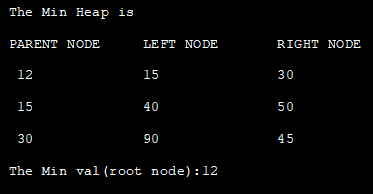### Max Heap In Java

A max heap is also a complete binary tree. In a max heap, the root node is greater than or equal to the child nodes. In general, the value of any internal node in a max heap is greater than or equal to its child nodes.

While max heap is mapped to an array, if any node is stored at position ‘i’, then its left child is stored at 2i +1 and the right child is stored at 2i + 2.

Typical Max-heap will look as shown below: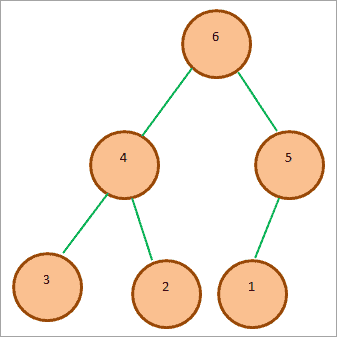In the above diagram, we see that the root node is the largest in heap and its child nodes have values smaller than the root node.

Similar to min-heap, the max heap can also be represented as an array.

So if A is an array that represents Max heap then A  is the root node. Similarly, if A[i] is any node in the max heap, then the following are the other adjacent nodes that can be represented using an array.

• A [(i-1)/2] represents the parent node of A[i].
• A [(2i +1)] represents the left child node of A[i].
• A [2i+2] returns the right child node of A[i].

The operations that can be performed on the Max Heap are given below.

#1) Insert: Insert operation inserts a new value in the max heap tree. It is inserted at the end of the tree. If the new key (value) is smaller than its parent node, then the heap property is maintained. Otherwise, the tree needs to be heapified to maintain the heap property.

The time complexity of insert operation is O (log n).

#2) ExtractMax: The operation ExtractMax removes the maximum element (root ) from the max heap. The operation also heapifies the max heap to maintain the heap property. The time complexity of this operation is O (log n).

#3) getMax: getMax operation returns the root node of the max heap with the time complexity of O (1).

The below Java program implements the max heap. We make use of ArrayList here to represent the max heap elements.

 `import` `java.util.ArrayList;``class` `Heap {``  ``void` `heapify(ArrayList hT, ``int` `i) {``    ``int` `size = hT.size();``    ``int` `largest = i;``    ``int` `l = ``2` `* i + ``1``;``    ``int` `r = ``2` `* i + ``2``;``    ``if` `(l < size && hT.get(l) > hT.get(largest))``      ``largest = l;``    ``if` `(r < size && hT.get(r) > hT.get(largest))``      ``largest = r;``    ``if` `(largest != i) {``      ``int` `temp = hT.get(largest);``      ``hT.set(largest, hT.get(i));``      ``hT.set(i, temp);``      ``heapify(hT, largest);``    ``}``  ``}``  ``void` `insert(ArrayList hT, ``int` `newNum) {``    ``int` `size = hT.size();``    ``if` `(size == ``0``) {``      ``hT.add(newNum);``    ``} ``else` `{``      ``hT.add(newNum);``      ``for` `(``int` `i = size / ``2` `- ``1``; i >= ``0``; i--) {``        ``heapify(hT, i);``      ``}``    ``}``  ``}``  ``void` `deleteNode(ArrayList hT, ``int` `num)   {``    ``int` `size = hT.size();``    ``int` `i;``    ``for` `(i = ``0``; i < size; i++) {``      ``if` `(num == hT.get(i))``        ``break``;``    ``}``    ``int` `temp = hT.get(i);``    ``hT.set(i, hT.get(size-``1``));``    ``hT.set(size-``1``, temp);``    ``hT.remove(size-``1``);``    ``for` `(``int` `j = size / ``2` `- ``1``; j >= ``0``; j--)  {``      ``heapify(hT, j);``    ``}``  ``}``  ``void` `printArray(ArrayList array, ``int` `size) {``    ``for` `(Integer i : array) {``      ``System.out.print(i + ``" "``);``    ``}``    ``System.out.println();``  ``}``}``class` `Main{``  ``public` `static` `void` `main(String args[]) {``    ``ArrayList array = ``new` `ArrayList();``    ``int` `size = array.size();``    ``Heap h = ``new` `Heap();``    ``h.insert(array, ``3``);``    ``h.insert(array, ``4``);``    ``h.insert(array, ``9``);``    ``h.insert(array, ``5``);``    ``h.insert(array, ``2``);``    ``System.out.println(``"Max-Heap array: "``);``    ``h.printArray(array, size);``    ``h.deleteNode(array, ``4``);``    ``System.out.println(``"After deleting an element: "``);``    ``h.printArray(array, size);``  ``}``}`

Output: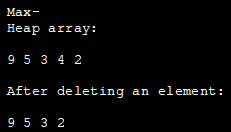### Priority Queue Min Heap In Java

The priority queue data structure in Java can be directly used to represent the min-heap. By default, the priority queue implements min-heap.

The below program demonstrates the min-heap in Java using the Priority Queue.

 `import` `java.util.*; ``  ``class` `Main {   ``    ``public` `static` `void` `main(String args[])  { ``        ``// Create priority queue object ``        ``PriorityQueue pQueue_heap = ``new` `PriorityQueue(); ``        ``// Add elements to the pQueue_heap using add() ``        ``pQueue_heap.add(``100``); ``        ``pQueue_heap.add(``30``); ``        ``pQueue_heap.add(``20``); ``        ``pQueue_heap.add(``40``); ``  ``        ``// Print the head (root node of min heap) using peek method ``        ``System.out.println(``"Head (root node of min heap):"` `+ pQueue_heap.peek()); ``        ``// Print min heap represented using PriorityQueue ``        ``System.out.println(``"\n\nMin heap as a PriorityQueue:"``); ``        ``Iterator iter = pQueue_heap.iterator(); ``        ``while` `(iter.hasNext()) ``            ``System.out.print(iter.next() + ``" "``); ``  ``        ``// remove head (root of min heap) using poll method ``        ``pQueue_heap.poll(); ``        ``System.out.println(``"\n\nMin heap after removing root node:"``); ``        ``//print the min heap again``        ``Iterator iter2 = pQueue_heap.iterator(); ``        ``while` `(iter2.hasNext()) ``            ``System.out.print(iter2.next() + ``" "``); ``    ``} ``}`

Output: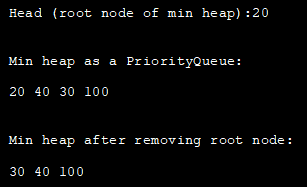### Priority Queue Max Heap In Java

To represent the max heap in Java using the Priority queue, we have to use Collections.reverseOrder to reverse the min-heap. The priority queue directly represents a min-heap in Java.

We have implemented the Max Heap using a Priority queue in the below program.

 `import` `java.util.*; ``  ``class` `Main { ``    ``public` `static` `void` `main(String args[])  { ``        ``// Create empty priority queue ``        ``//with Collections.reverseOrder to represent max heap``        ``PriorityQueue pQueue_heap =  ``             ``new` `PriorityQueue(Collections.reverseOrder()); ``  ``        ``// Add items to the pQueue using add() ``        ``pQueue_heap.add(``10``); ``        ``pQueue_heap.add(``90``); ``        ``pQueue_heap.add(``20``); ``        ``pQueue_heap.add(``40``); ``  ``        ``// Printing all elements of max heap ``        ``System.out.println(``"The max heap represented as PriorityQueue:"``); ``        ``Iterator iter = pQueue_heap.iterator(); ``        ``while` `(iter.hasNext()) ``            ``System.out.print(iter.next() + ``" "``); ``  ``        ``// Print the highest priority element (root of max heap) ``        ``System.out.println(``"\n\nHead value (root node of max heap):"` `+ ``                                              ``pQueue_heap.peek()); ``        ``        ``// remove head (root node of max heap) with poll method ``        ``pQueue_heap.poll(); ``        ``//print the max heap again``        ``System.out.println(``"\n\nMax heap after removing root: "``); ``        ``Iterator iter2 = pQueue_heap.iterator(); ``        ``while` `(iter2.hasNext()) ``            ``System.out.print(iter2.next() + ``" "``); ``      ``} ``}`

Output: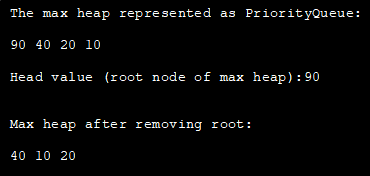### Heap Sort In Java

Heap sort is a comparison sort technique similar to selection sort wherein we select a maximum element in the array for each iteration. Heap sort makes use of Heap data structure and sorts the elements by creating min or max heap out of the array elements to be sorted.

We have already discussed that in min and max heap, the root node contains the minimum and maximum element respectively of the array. In heap sort, the root element of the heap (min or max) is removed and moved to the sorted array. The remaining heap is then heapified to maintain the heap property.

So we have to perform two steps recursively to sort the given array using heap sort.

• Build a heap from the given array.
• Repeatedly remove the root element from the heap and move it to the sorted array. Heapify the remaining heap.

Time Complexity of Heap sort is O (n log n) in all the cases. The space complexity is O (1).

#### Heap Sort Algorithm In Java

Given below are the heap sort algorithms to sort the given array in ascending and descending order.

#1) Heap Sort algorithm to sort in ascending order:

• Create a max heap for the given array to be sorted.
• Delete the root (maximum value in the input array) and move it to the sorted array. Place the last element in the array at the root.
• Heapify the new root of the heap.
• Repeat steps 1 and 2 till the entire array is sorted.

#2) Heap Sort algorithm to sort in descending order:

• Construct a min Heap for the given array.
• Remove the root (minimum value in the array) and swap it with the last element in the array.
• Heapify the new root of the heap.
• Repeat steps 1 and 2 till the entire array is sorted.

#### Heap Sort Implementation In Java

The below Java program uses heap sort to sort an array in ascending order. For this, we first construct a max heap and then recursively swap and heapify the root element as specified in the above algorithm.

 `import` `java.util.*;``class` `HeapSort{``      ``public` `void` `heap_sort(``int` `heap_Array[]) {``      ``int` `heap_len = heap_Array.length;``      ``// construct max heap``      ``for` `(``int` `i = heap_len / ``2` `- ``1``; i >= ``0``; i--) {``        ``heapify(heap_Array, heap_len, i);``      ``}``      ``// Heap sort``      ``for` `(``int` `i = heap_len - ``1``; i >= ``0``; i--) {``        ``int` `temp = heap_Array[``0``];``        ``heap_Array[``0``] = heap_Array[i];``        ``heap_Array[i] = temp;``        ``// Heapify root element``        ``heapify(heap_Array, i, ``0``);``      ``}``    ``}``  ``    ``void` `heapify(``int` `heap_Array[], ``int` `n, ``int` `i) {``      ``// find largest value``      ``int` `largest = i;``      ``int` `left = ``2` `* i + ``1``;``      ``int` `right = ``2` `* i + ``2``;``      ``if` `(left < n && heap_Array[left] > heap_Array[largest])``     ``largest = left;``      ``if` `(right < n && heap_Array[right] > heap_Array[largest])``        ``largest = right;``      ``// recursively heapify and swap if root is not the largest``      ``if` `(largest != i) {``        ``int` `swap = heap_Array[i];``        ``heap_Array[i] = heap_Array[largest];``        ``heap_Array[largest] = swap;``        ``heapify(heap_Array, n, largest);``      ``}``    ``}``}``class` `Main{`` ``public` `static` `void` `main(String args[]) {``        ``//define input array and print it``        ``int` `heap_Array[] = {``6``,``2``,``9``,``4``,``10``,``15``,``1``,``13``};``        ``System.out.println(``"Input Array:"` `+ Arrays.toString(heap_Array));``        ``//call HeapSort method for given array``        ``HeapSort hs = ``new` `HeapSort();``        ``hs.heap_sort(heap_Array);``        ``//print the sorted array  ``        ``System.out.println(``"Sorted Array:"` `+ Arrays.toString(heap_Array));``    ``}``}`

Output: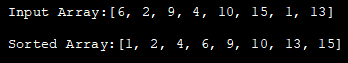The overall time complexity of the heap sort technique is O (nlogn). The time complexity of the heapify technique is O (logn). While the time complexity of building the heap is O (n).

## Stack Vs Heap In Java

Let’s now tabularize some of the differences between a Stack data structure and heap.

StackHeap
A stack is a linear data structure.A heap is a hierarchical data structure.
Follows LIFO (Last In, First Out) ordering.Traversal is in level order.
Mostly used for static memory allocation.Used for dynamic memory allocation.
Memory is allocated contiguously.Memory is allocated in random locations.
Stack size is limited according to the Operating system.No limit on heap size enforced by the Operating system.
Stack has access to local variables only.Heap has global variables allocated to it.
Access is faster.Slower than the stack.
The allocation/deallocation of memory is automatic.Allocation/deallocation needs to be done manually by the programmer.
The stack can be implemented using Arrays, Linked List, ArrayList, etc. or any other linear data structures.Heap is implemented using Arrays or trees.
Cost of maintenance if less.More costly to maintain.
May result in a shortage of memory as memory is limited.No shortage of memory but may suffer from memory fragmentation.

### Frequently Asked Questions

Q #1) Is stack faster than Heap?

Answer: A stack is faster than heap as access is linear in stack compared to the heap.

Q #2) What is a Heap used for?

Answer: Heap is mostly used in algorithms that find the minimum or shortest path between two points like Dijkstra’s algorithm, to sort using heap sort, for priority queue implementations (min-heap), etc.

Q #3) What is a Heap? What are its types?

Answer: A heap is a hierarchical, tree-based data structure. A heap is a complete binary tree. Heaps are of two types i.e. Max heap in which the root node is the largest among all the nodes; Min heap in which the root node is the smallest or minimum among all the keys.

Q #4) What are the advantages of Heap over a stack?

Answer: The major advantage of the heap over stack is in heap, the memory is dynamically allocated and hence there is no limit on how much memory can be used. Secondly, only local variables can be allocated on the stack while we can also allocate global variables on the heap.

Q #5) Can Heap have duplicates?

Answer: Yes, there are no restrictions on having nodes with duplicate keys in the heap as the heap is a complete binary tree and it does not satisfy the properties of the binary search tree.

## Conclusion

In this instructional exercise, we have talked about the kinds of store and load sort utilizing kinds of stack. We have likewise seen the definite execution of its sorts in Java.